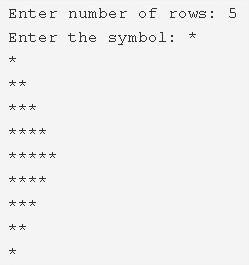# C Program Half Diamond Star Pattern | C Pattern Programs

C program to print a half diamond star pattern – In this article, we will brief in on the several methods used to print a half diamond star pattern in C programming.

Suitable examples and sample programs have also been added so that you can understand the whole thing very clearly. The compiler has also been added with which you can execute it yourself.

The ways used to do so in this specific article are as follows:

• Using For Loop
• Using While Loop
• Using Do-While Loop

As we all know, a diamond is a very popular shape that is generally used in daily life, unlike the actual thing which is extremely expensive.

This is how a half diamond star pattern looks like:As you can see, firstly, you need to enter the number of rows to print the diamond star.

Then, the number that you have entered, those many rows will be printed on both sides of the middle.

Thus, the ways to print a half diamond star pattern are as follows:

## Using For Loop

1. Read the no.of rows, symbol and store the rows number into n, symbol into ch.

2) The 1st for loop iterates through rows with the structure for(i=1;i<=n;i++).

a) The 2nd for loop iterates through columns with the structure for(j=1;j<=i;j++).

It prints symbol.

b) Cursor comes to next line after each iteration of the 1st for the loop.

An upper part of the half diamond pattern will be formed after all iterations of above for loops.

3) The 3rd for loop iterates through rows with the structure for(i=n-1;i>0;i–).

a) The 4th for loop iterates through columns with the structure for(j=1;j<=i;j++)

It prints symbol.

b) Cursor comes to next line for each iteration of the  3rd for the loop.

A lower part of the half diamond will be formed after all iterations of 3rd for loop, 4th for the loop.

Output:

### Using While Loop

1. The 1st while loop iterates through rows until the condition i<=n becomes false.

a)The 2nd while loop iterates through columns until the condition j<=i becomes false.

It prints symbol.j value increased by 1.2nd while loop again checks the condition.

b) Cursor comes to next line.i value increased by 1. The 1st while loop again checks the condition.

2,3 steps represent the upper part of the half diamond star pattern.

2) i=n-1, The 3rd while loop iterates through rows until the condition i>0 becomes false.

a) The 4th while loop iterates through columns until the condition j<=i becomes false.

It prints symbol.j value increased by 1. The 4th while loop again checks the condition.

b) Cursor comes to next line. i value decreased by 1. The 3rd while loop again checks the condition.

Output:

#### Using Do-While Loop

1. For half part of the half diamond star pattern.

2) The 1st do-while loop iterates through rows.

a) The 2nd do-while loop iterates through columns.

It prints symbol.j value increased by 1.

Checks the condition j<=i. If condition true then loop iterates again. Repeats until the condition becomes false.

b) Cursor comes to next line.i value increased by 1.

c) Checks the condition i<=n. If the condition is true then the loop iterates again. Repeats until the condition becomes false.

For below half part of the half diamond star pattern.

3)i=n-1, The 3rd do-while loop iterates through rows.

a) j=1, The 4th do-while loop iterates through columns.

It prints symbol. j value increased by 1.

Checks the condition j<=i. If the condition is true then loop iterates again. Repeats until the condition becomes false.

b) Cursor comes to next line.i value decreased by 1.

c) Checks the condition i>0. If the condition is true, loop iterates again. Repeats until the condition becomes false.

Output:
x

## C Program Inverted Pyramid Star Pattern | 4 Ways – C Programs

C Program to print an inverted pyramid star pattern – In this article, we will ...